Topics: AI | Data Science | Data Visualization | Deep Learning | Machine Learning | NLP | Python | R | Statistics

KDnuggets Home » News » 2017 » Oct » Tutorials, Overviews » 7 Types of Artificial Neural Networks for Natural Language Processing

#7 Types of Artificial Neural Networks for Natural Language Processing

What is an artificial neural network? How does it work? What types of artificial neural networks exist? How are different types of artificial neural networks used in natural language processing? We will discuss all these questions in the following article.

By Olga Davydova, Data Monsters.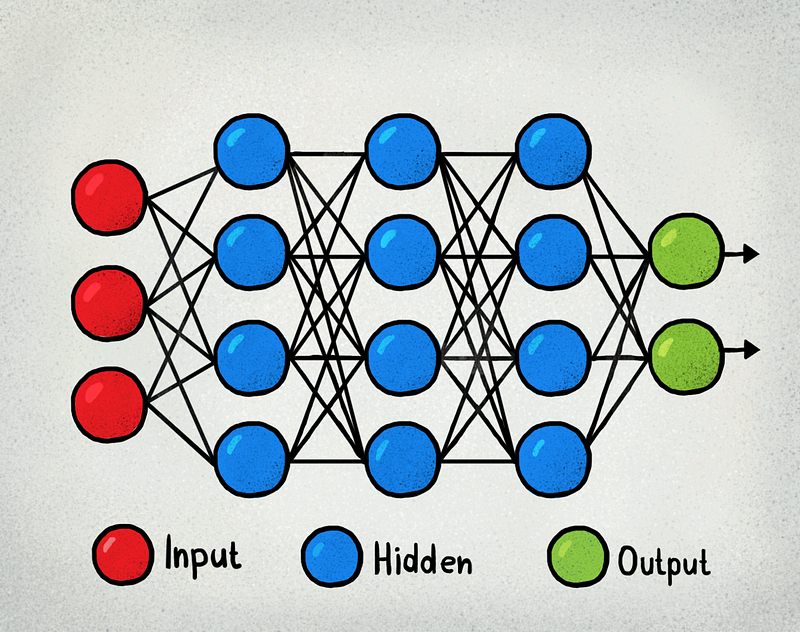An artificial neural network (ANN) is a computational nonlinear model based on the neural structure of the brain that is able to learn to perform tasks like classification, prediction, decision-making, visualization, and others just by considering examples.

An artificial neural network consists of artificial neurons or processing elements and is organized in three interconnected layers: input, hidden that may include more than one layer, and output.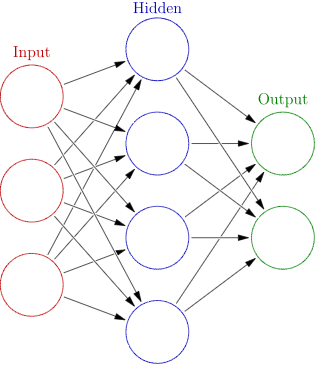An artificial neural network (https://en.wikipedia.org/wiki/Artificial_neural_network#/media/File:Colored_neural_network.svg)

The input layer contains input neurons that send information to the hidden layer. The hidden layer sends data to the output layer. Every neuron has weighted inputs (synapses), an activation function (defines the output given an input), and one output. Synapses are the adjustable parameters that convert a neural network to a parameterized system.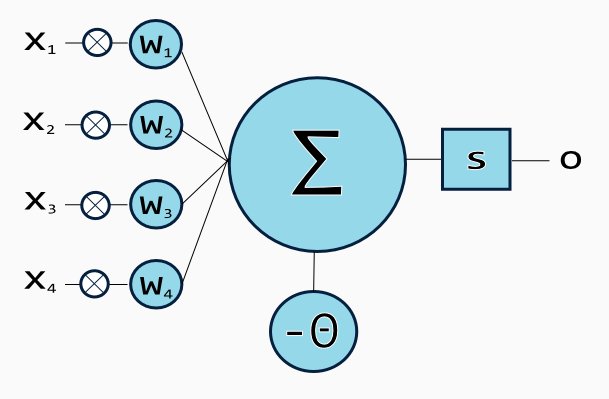Artificial neuron with four inputs (http://en.citizendium.org/wiki/File:Artificialneuron.png)

The weighted sum of the inputs produces the activation signal that is passed to the activation function to obtain one output from the neuron. The commonly used activation functions are linear, step, sigmoid, tanh, and rectified linear unit (ReLu) functions.

Linear Function

f(x)=ax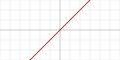Step Function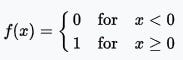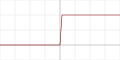Logistic (Sigmoid) Function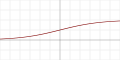Tanh Function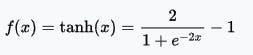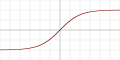Rectified linear unit (ReLu) Function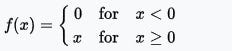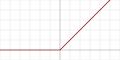Training is the weights optimizing process in which the error of predictions is minimized and the network reaches a specified level of accuracy. The method mostly used to determine the error contribution of each neuron is called backpropagation that calculates the gradient of the loss function.

It is possible to make the system more flexible and more powerful by using additional hidden layers. Artificial neural networks with multiple hidden layers between the input and output layers are called deep neural networks (DNNs), and they can model complex nonlinear relationships.

### 1. Multilayer perceptron (MLP)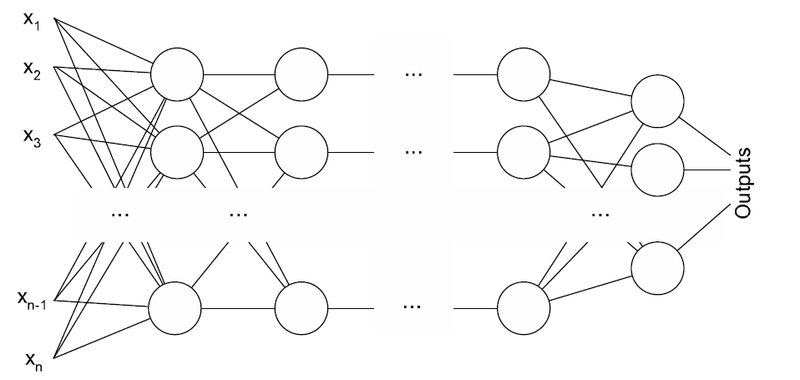A multilayer perceptron (MLP) has three or more layers. It utilizes a nonlinear activation function (mainly hyperbolic tangent or logistic function) that lets it classify data that is not linearly separable. Every node in a layer connects to each node in the following layer making the network fully connected. For example, multilayer perceptron natural language processing (NLP) applications are speech recognition and machine translation.

### 2. Convolutional neural network (CNN)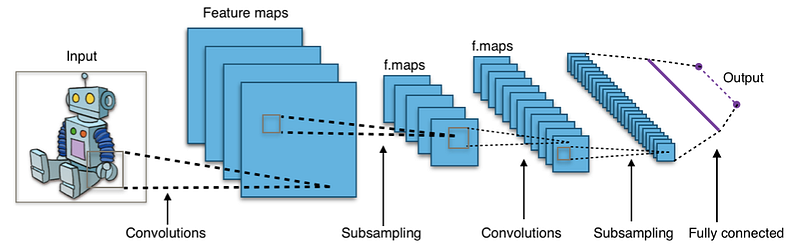Typical CNN architecture (https://en.wikipedia.org/wiki/Convolutional_neural_network#/media/File:Typical_cnn.png)

A convolutional neural network (CNN) contains one or more convolutional layers, pooling or fully connected, and uses a variation of multilayer perceptrons discussed above. Convolutional layers use a convolution operation to the input passing the result to the next layer. This operation allows the network to be deeper with much fewer parameters.

Convolutional neural networks show outstanding results in image and speech applications. Yoon Kim in Convolutional Neural Networks for Sentence Classification describes the process and the results of text classification tasks using CNNs . He presents a model built on top of word2vec, conducts a series of experiments with it, and tests it against several benchmarks, demonstrating that the model performs excellent.

In Text Understanding from Scratch, Xiang Zhang and Yann LeCun, demonstrate that CNNs can achieve outstanding performance without the knowledge of words, phrases, sentences and any other syntactic or semantic structures with regards to a human language . Semantic parsing , paraphrase detection , speech recognition  are also the applications of CNNs.Get KDnuggets, a leading newsletter on AI, Data Science, and Machine Learning